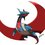# Factoring quartics-2 special cases!

part-1. i am going to show you some special of a quartic polynomial which makes it easier to solve. in future notes i will try to generalize for every quartic polynomials. 2 cases to be presented here:

case 1: consider the polynomial $f(x)=x^4+ax^3+bx^2+acx+c^2$ i think most of you know how to factor this easily, but i am going to continue regardless. $\begin{array}{c}a f(x)=x^2(x^2+ax+b+acx^{-1}+c^2x^{-2})\\ \rightarrow x^2+2+(cx^{-1})^2+a(x+cx^{-1})+b-2=0\\ (x+cx^{-1})^2+a(x+cx^{-1})+b-2=0\\ x+cx^{-1}=\dfrac{-a\pm\sqrt{a^2-4b+8}}{2}\\ x^2-\dfrac{-a\pm\sqrt{a^2-4b+8}}{2}x+c=0\end{array}$ i think this is the most general form, as going farther will simply make it more tedious.  case 2 $x^4-2ax^2-x+a^2-a=0$ $x^4-2ax^2+a^2=a+x$ $x^2-a=\pm\sqrt{a+x}$ $x=\pm\sqrt{a+\sqrt{a+x}},\pm\sqrt{a-\sqrt{a+x}}$ first one $x=\sqrt{a+\sqrt{a+x}}=\sqrt{a+\sqrt{a+\sqrt{a+\sqrt{a+x}}}}=\sqrt{a+\sqrt{a+...}}=\sqrt{a+x}$ $x^2-x+a=0$ the minus sign will have the negative root, and the positive sign the positive. second one: $x=\sqrt{a-\sqrt{a+\sqrt{a-\sqrt{a+...}}}},y=\sqrt{a+\sqrt{a-\sqrt{a+\sqrt{a-...}}}}$ $x^2=a-y,y^2=a+x$ subtract both $x^2-y^2=-y-x\Longrightarrow x-y=-1$ $x^2=a-(x+1)\Longrightarrow x^2+x+(1-a)=0$ we can actually simplify these two results to get our original polynomials.Note by Aareyan Manzoor
5 years, 6 months ago

This discussion board is a place to discuss our Daily Challenges and the math and science related to those challenges. Explanations are more than just a solution — they should explain the steps and thinking strategies that you used to obtain the solution. Comments should further the discussion of math and science.

When posting on Brilliant:

• Use the emojis to react to an explanation, whether you're congratulating a job well done , or just really confused .
• Ask specific questions about the challenge or the steps in somebody's explanation. Well-posed questions can add a lot to the discussion, but posting "I don't understand!" doesn't help anyone.
• Try to contribute something new to the discussion, whether it is an extension, generalization or other idea related to the challenge.
• Stay on topic — we're all here to learn more about math and science, not to hear about your favorite get-rich-quick scheme or current world events.

MarkdownAppears as
*italics* or _italics_ italics
**bold** or __bold__ bold
- bulleted- list
• bulleted
• list
1. numbered2. list
1. numbered
2. list
Note: you must add a full line of space before and after lists for them to show up correctly
paragraph 1paragraph 2

paragraph 1

paragraph 2

[example link](https://brilliant.org)example link
> This is a quote
This is a quote
    # I indented these lines
# 4 spaces, and now they show
# up as a code block.

print "hello world"
# I indented these lines
# 4 spaces, and now they show
# up as a code block.

print "hello world"
MathAppears as
Remember to wrap math in $$ ... $$ or $ ... $ to ensure proper formatting.
2 \times 3 $2 \times 3$
2^{34} $2^{34}$
a_{i-1} $a_{i-1}$
\frac{2}{3} $\frac{2}{3}$
\sqrt{2} $\sqrt{2}$
\sum_{i=1}^3 $\sum_{i=1}^3$
\sin \theta $\sin \theta$
\boxed{123} $\boxed{123}$

## Comments

Sort by:

Top Newest

Did'nt understand •_

- 5 years, 5 months ago

Log in to reply

×

Problem Loading...

Note Loading...

Set Loading...